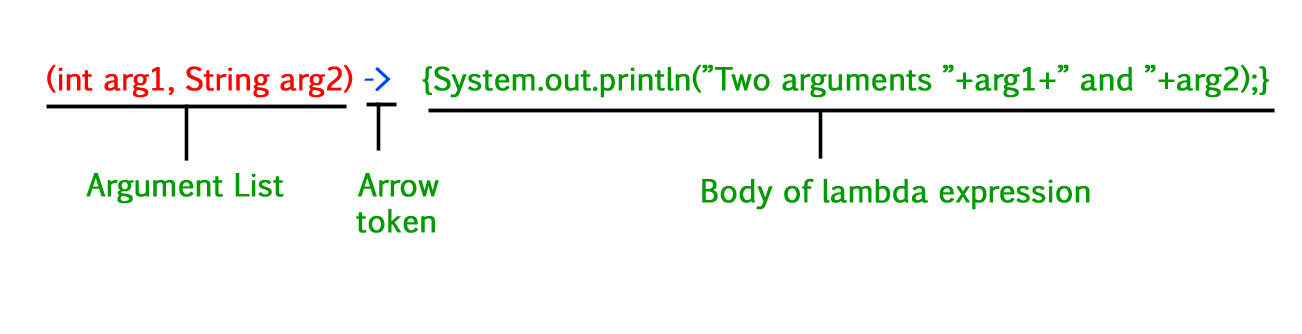Open In App
Related Articles

# Lambda Expression in Java

In Java, Lambda expressions basically express instances of functional interfaces (An interface with a single abstract method is called a functional interface). Lambda Expressions in Java are the same as lambda functions which are the short block of code that accepts input as parameters and returns a resultant value. Lambda Expressions are recently included in Java SE 8.

## Functionalities of Lambda Expression in Java

Lambda Expressions implement the only abstract function and therefore implement functional interfaces lambda expressions are added in Java 8 and provide the below functionalities.

• Enable to treat functionality as a method argument, or code as data.
• A function that can be created without belonging to any class.
• A lambda expression can be passed around as if it was an object and executed on demand.

## Java

 `// Java program to demonstrate lambda expressions``// to implement a user defined functional interface.` `// A sample functional interface (An interface with``// single abstract method``interface` `FuncInterface``{``    ``// An abstract function``    ``void` `abstractFun(``int` `x);` `    ``// A non-abstract (or default) function``    ``default` `void` `normalFun()``    ``{``       ``System.out.println(``"Hello"``);``    ``}``}` `class` `Test``{``    ``public` `static` `void` `main(String args[])``    ``{``        ``// lambda expression to implement above``        ``// functional interface. This interface``        ``// by default implements abstractFun()``        ``FuncInterface fobj = (``int` `x)->System.out.println(``2``*x);` `        ``// This calls above lambda expression and prints 10.``        ``fobj.abstractFun(``5``);``    ``}``}`

Output

```10

```

##Lambda Expression Syntax

` lambda operator -> body`

### Lambda Expression Parameters

There are three Lambda Expression Parameters are mentioned below:

1. Zero Parameter
2. Single Parameter
3. Multiple Parameters

### 1. Lambda Expression with Zero parameter

`() -> System.out.println("Zero parameter lambda");`

### 2. Lambda Expression with Single parameter

`(p) -> System.out.println("One parameter: " + p);`

It is not mandatory to use parentheses if the type of that variable can be inferred from the context

## Java

 `// A Java program to demonstrate simple lambda expressions``import` `java.util.ArrayList;``class` `Test {``    ``public` `static` `void` `main(String args[])``    ``{``        ``// Creating an ArrayList with elements``        ``// {1, 2, 3, 4}``        ``ArrayList arrL = ``new` `ArrayList();``        ``arrL.add(``1``);``        ``arrL.add(``2``);``        ``arrL.add(``3``);``        ``arrL.add(``4``);` `        ``// Using lambda expression to print all elements``        ``// of arrL``        ``arrL.forEach(n -> System.out.println(n));` `        ``// Using lambda expression to print even elements``        ``// of arrL``        ``arrL.forEach(n -> {``            ``if` `(n % ``2` `== ``0``)``                ``System.out.println(n);``        ``});``    ``}``}`

Output

```1
2
3
4
2
4

```

Note: that lambda expressions can only be used to implement functional interfaces. In the above example also, the lambda expression implements Consumer Functional Interface.

### 3. Lambda Expression with Multiple parameters

`(p1, p2) -> System.out.println("Multiple parameters: " + p1 + ", " + p2);`

A Java program to demonstrate the working of a lambda expression with two arguments.

## Java

 `// Java program to demonstrate working of lambda expressions``public` `class` `Test {``    ``// operation is implemented using lambda expressions``    ``interface` `FuncInter1 {``        ``int` `operation(``int` `a, ``int` `b);``    ``}` `    ``// sayMessage() is implemented using lambda expressions``    ``// above``    ``interface` `FuncInter2 {``        ``void` `sayMessage(String message);``    ``}` `    ``// Performs FuncInter1's operation on 'a' and 'b'``    ``private` `int` `operate(``int` `a, ``int` `b, FuncInter1 fobj)``    ``{``        ``return` `fobj.operation(a, b);``    ``}` `    ``public` `static` `void` `main(String args[])``    ``{``        ``// lambda expression for addition for two parameters``        ``// data type for x and y is optional.``        ``// This expression implements 'FuncInter1' interface``        ``FuncInter1 add = (``int` `x, ``int` `y) -> x + y;` `        ``// lambda expression multiplication for two``        ``// parameters This expression also implements``        ``// 'FuncInter1' interface``        ``FuncInter1 multiply = (``int` `x, ``int` `y) -> x * y;` `        ``// Creating an object of Test to call operate using``        ``// different implementations using lambda``        ``// Expressions``        ``Test tobj = ``new` `Test();` `        ``// Add two numbers using lambda expression``        ``System.out.println(``"Addition is "``                           ``+ tobj.operate(``6``, ``3``, add));` `        ``// Multiply two numbers using lambda expression``        ``System.out.println(``"Multiplication is "``                           ``+ tobj.operate(``6``, ``3``, multiply));` `        ``// lambda expression for single parameter``        ``// This expression implements 'FuncInter2' interface``        ``FuncInter2 fobj = message``            ``-> System.out.println(``"Hello "` `+ message);``        ``fobj.sayMessage(``"Geek"``);``    ``}``}`

Output

```Addition is 9
Multiplication is 18
Hello Geek

```

Note: Lambda expressions are just like functions and they accept parameters just like functions.

## Conclusion

• The body of a lambda expression can contain zero, one, or more statements.
• When there is a single statement curly brackets are not mandatory and the return type of the anonymous function is the same as that of the body expression.
• When there is more than one statement, then these must be enclosed in curly brackets (a code block) and the return type of the anonymous function is the same as the type of the value returned within the code block, or void if nothing is returned.

## FAQs in Lambda Expression

### Q1. What type of lambda expression Java?

Java Lambda Expressions are the short block of code that accepts input as parameters and returns a resultant value.

### Q2. Is it good to use lambda expressions in Java?

Yes, using lambda expressions makes it easier to use and support other APIs.

### Q3. What are the drawbacks of Java lambda?

Java lambda functions can be only used with functional interfaces.

### Q4. Based on the syntax rules just shown, which of the following is/are NOT valid lambda expressions?

1. () -> {}
2. () -> “geeksforgeeks”
3. () -> { return “geeksforgeeks”;)
4. (Integer i) -> return “geeksforgeeks” + i;
5. (String s) -> {“geeksforgeeks”;}